Скачать презентацию Complex Numbers Definition of pure imaginary numbers

• Количество слайдов: 19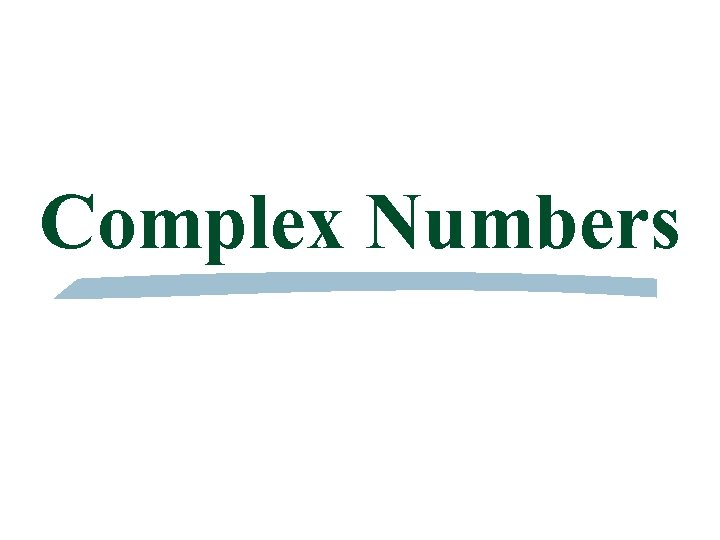Complex Numbers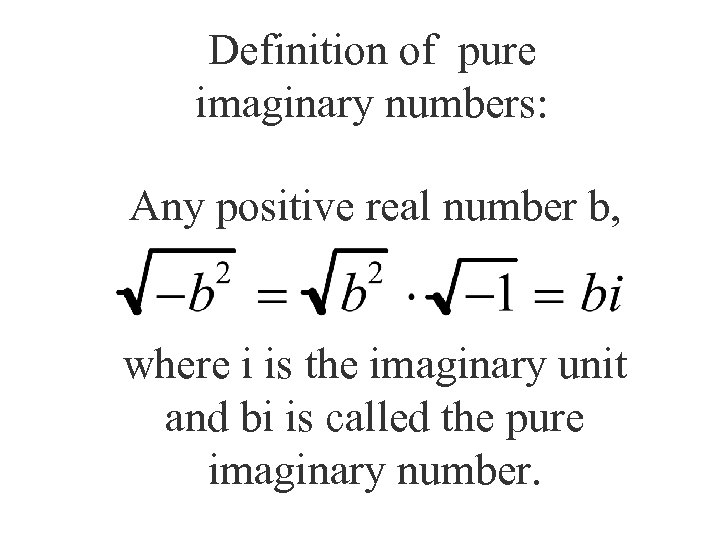Definition of pure imaginary numbers: Any positive real number b, where i is the imaginary unit and bi is called the pure imaginary number.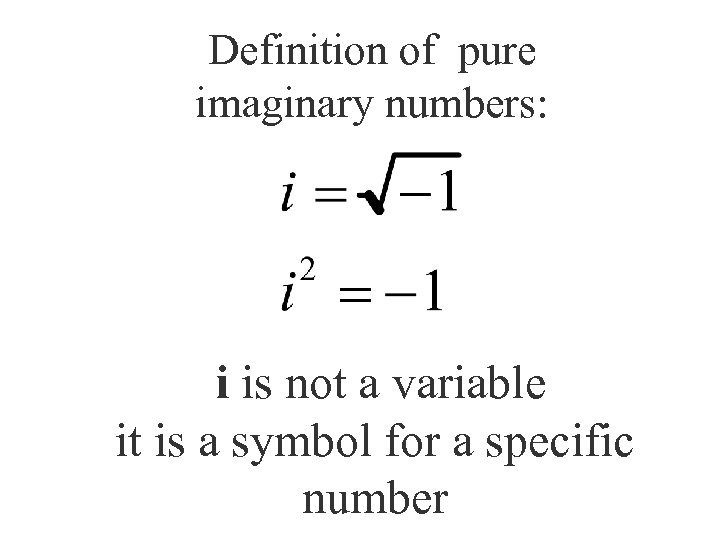Definition of pure imaginary numbers: i is not a variable it is a symbol for a specific number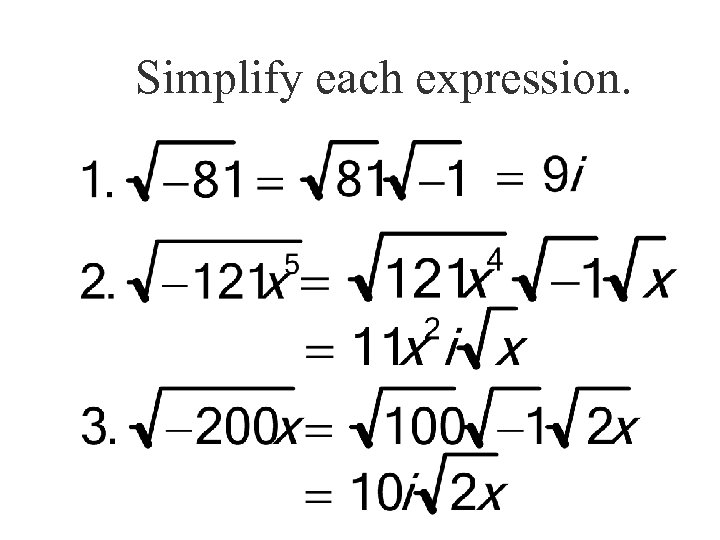Simplify each expression.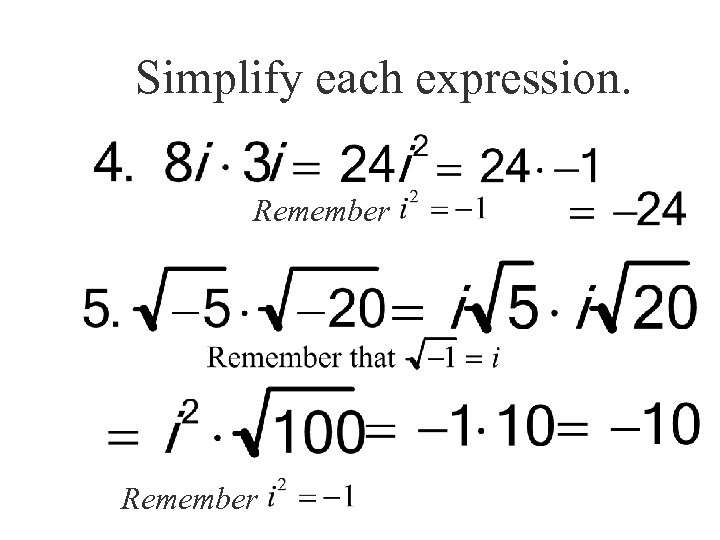Simplify each expression. Remember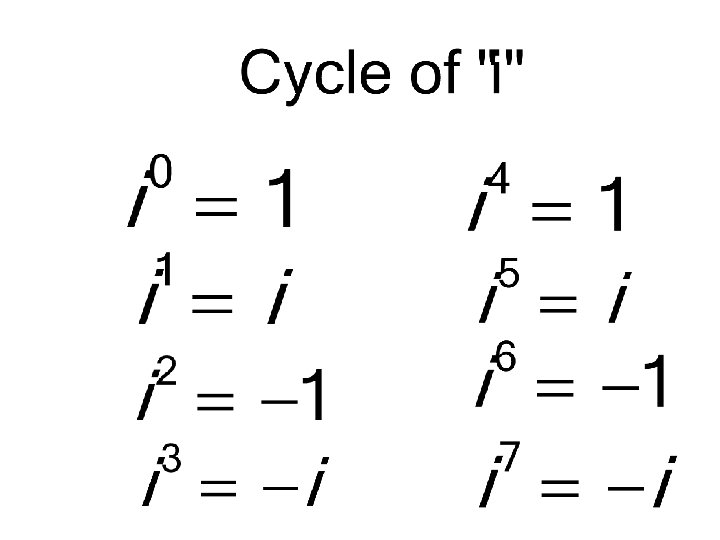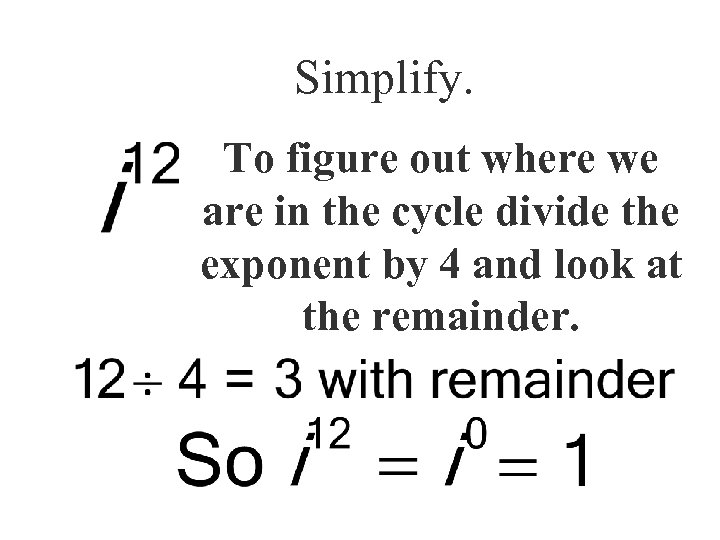Simplify. To figure out where we are in the cycle divide the exponent by 4 and look at the remainder.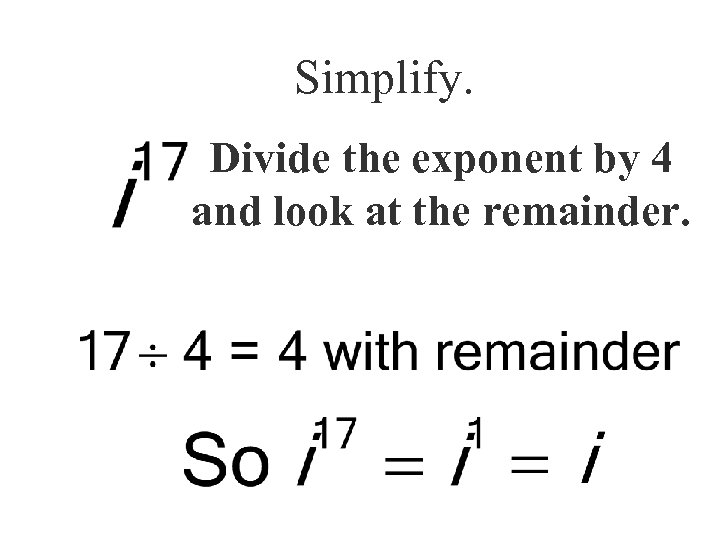Simplify. Divide the exponent by 4 and look at the remainder.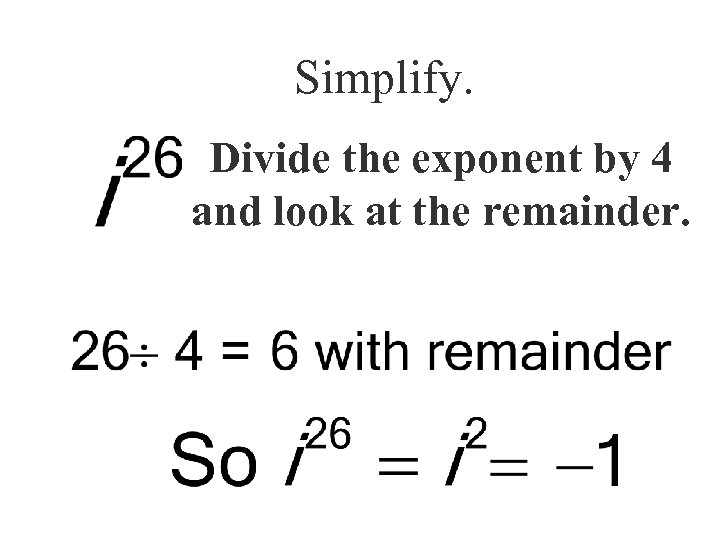Simplify. Divide the exponent by 4 and look at the remainder.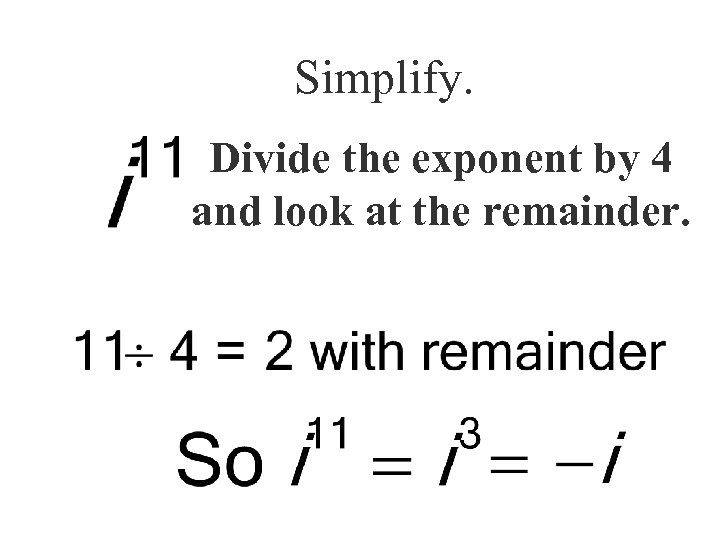Simplify. Divide the exponent by 4 and look at the remainder.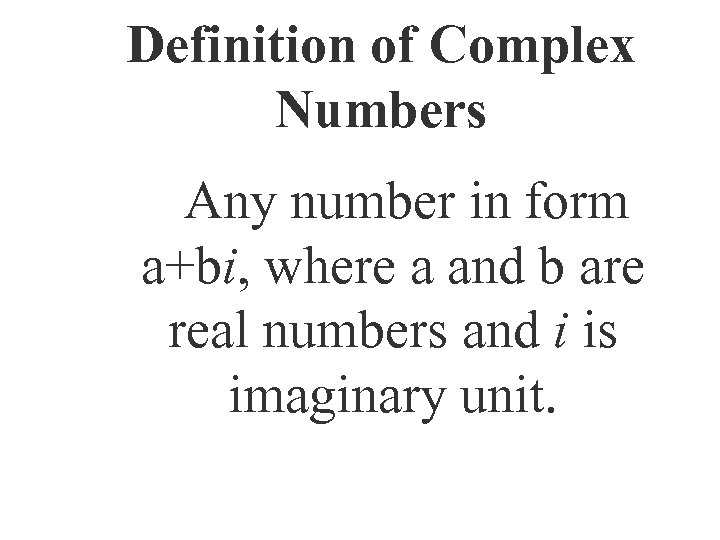Definition of Complex Numbers Any number in form a+bi, where a and b are real numbers and i is imaginary unit.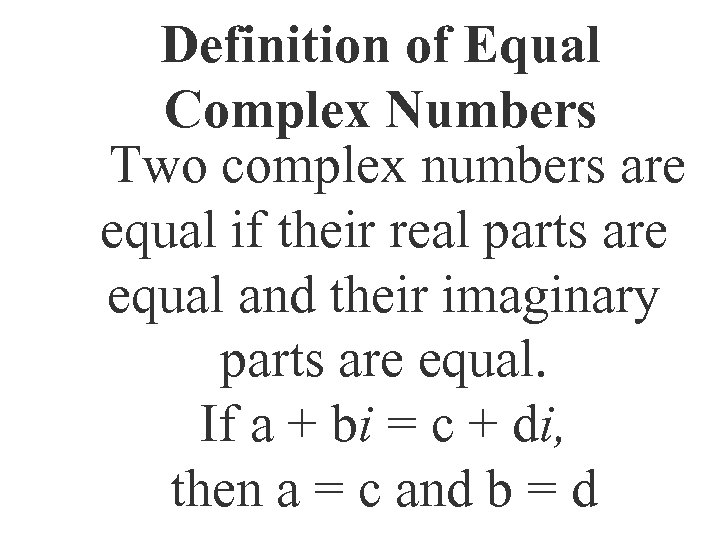Definition of Equal Complex Numbers Two complex numbers are equal if their real parts are equal and their imaginary parts are equal. If a + bi = c + di, then a = c and b = d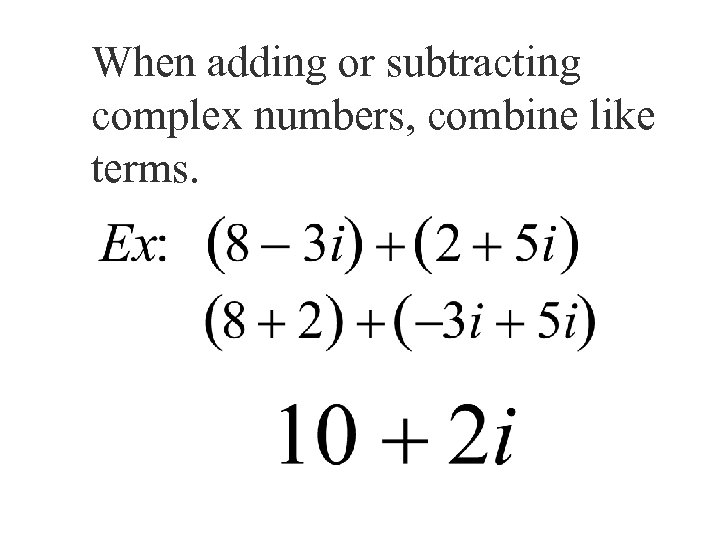When adding or subtracting complex numbers, combine like terms.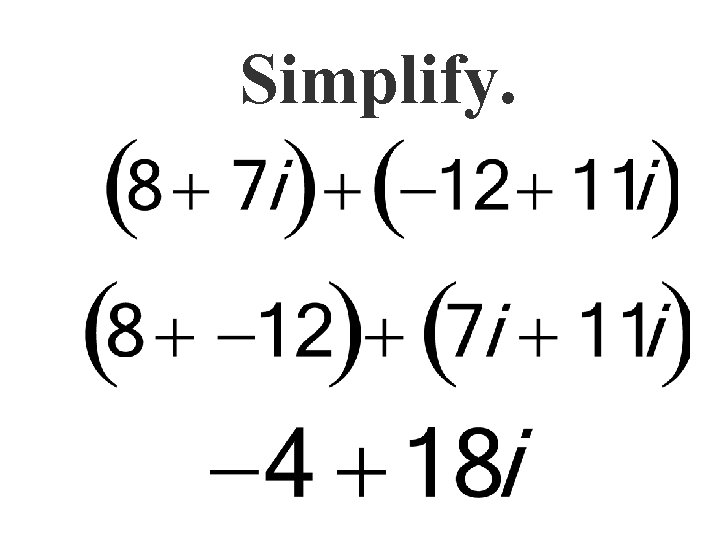Simplify.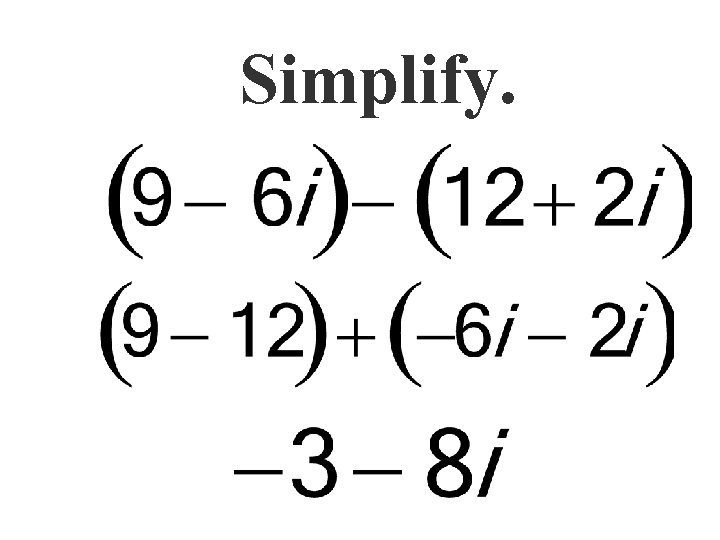Simplify.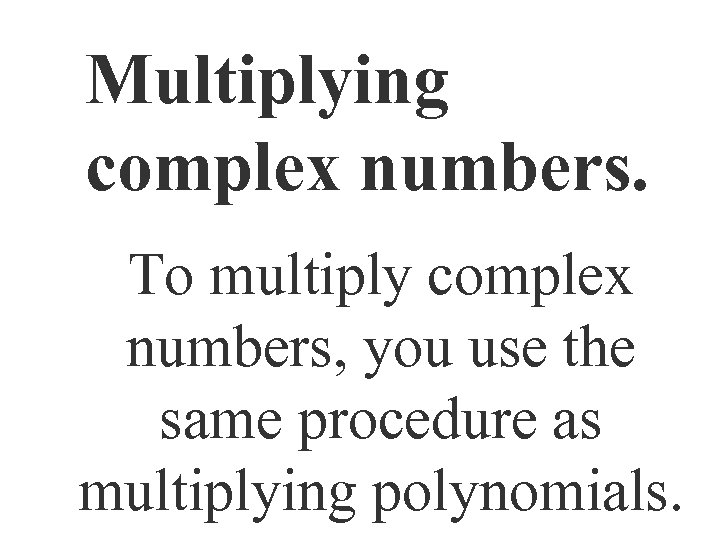Multiplying complex numbers. To multiply complex numbers, you use the same procedure as multiplying polynomials.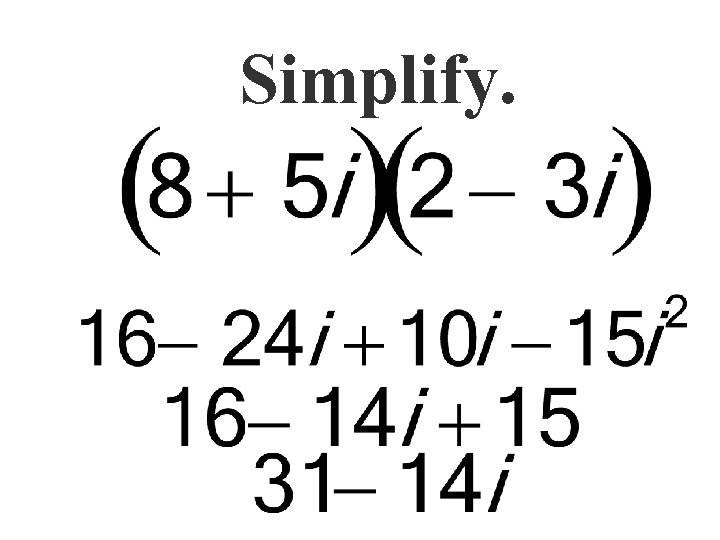Simplify.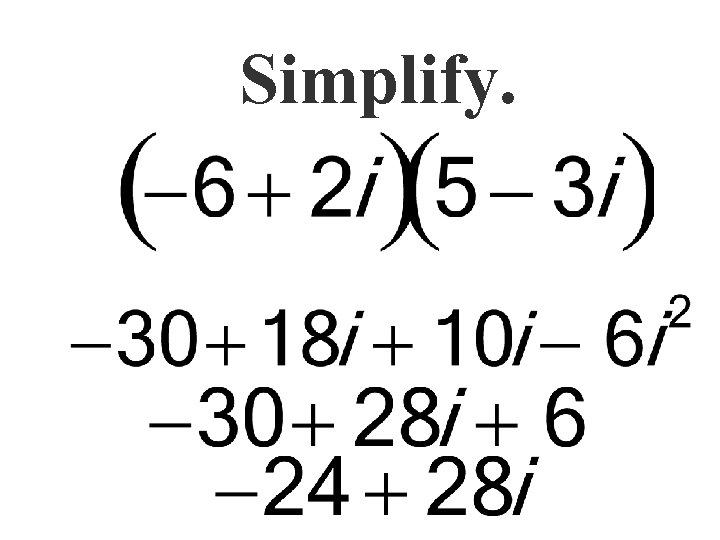Simplify.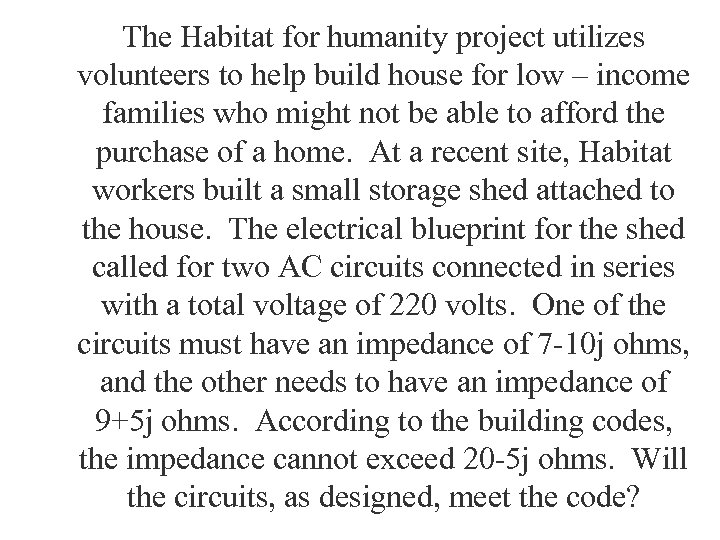The Habitat for humanity project utilizes volunteers to help build house for low – income families who might not be able to afford the purchase of a home. At a recent site, Habitat workers built a small storage shed attached to the house. The electrical blueprint for the shed called for two AC circuits connected in series with a total voltage of 220 volts. One of the circuits must have an impedance of 7 -10 j ohms, and the other needs to have an impedance of 9+5 j ohms. According to the building codes, the impedance cannot exceed 20 -5 j ohms. Will the circuits, as designed, meet the code?